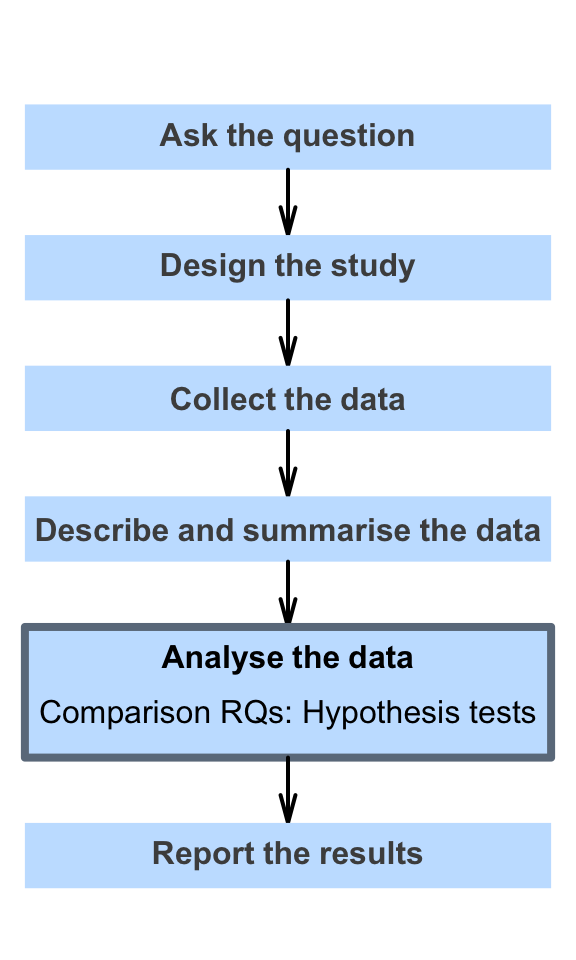# 26 Introducing hypothesis testsWe have studied forming confidence intervals, which answer estimation-type RQs, and indicate the precision with which a statistic estimates a parameter.

Now, we begin looking at decision-type RQs, which help us make decisions about the value of unknown parameters based on the value of the statistic (Table 26.1). The decision-making process (Chap. 15) we discussed was:

1. Assumption: Make an assumption about the population.

2. Expectation: Based on this assumption, the distribution of the possible values of the sample statistic can be described.

3. Observation: If sample information is observed that is:

• unlikely to happen by chance, it is contrary to that assumption about the population parameter, and the assumption is probably wrong. There is evidence to suggest that the assumption is wrong (but it is not certainly wrong).
• likely to happen by chance, it is consistent with that assumption about the population parameter, and the assumption may be correct. There is no evidence to suggest the assumption is wrong (though it may be wrong).

In this Part, we explore decision-type relational or interventional RQs with a comparison. (Decision-type RQs with a connection are discussed in Chaps. 34 and 35.)

TABLE 26.1: Confidence interrvals and hypothesis tests for different situations
Estimation type (CI) Decision type (Tests)
Descriptive RQs
Proportions for one sample Chap. 20
Means for one sample Chap. 22 Chap. 27
Mean differences (for paired data) Chap. 23 Chap. 29
Relational/Interventional RQs (Comparison)
Means for two samples Chap. 24 Chap. 30
Odds for two samples (ORs) Chap. 25 Chap. 31
Relational/Interventional RQs (Connection)
Correlation Sect. 34.4
Regression Sect. 35.7 Sect. 35.7